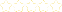• > Mary Merza
• > Solving equations with variables
+# Solving equations with variables

Owner: Mary Merza
##### Description:
How to simplify and solve equations

### Your Quiz Points in this Playlist

Total Possible
0
12 Tutorials in this Playlist

#### Combining Like Terms

Rating:(41)
This tutorial has a quiz
•• -

#### Simplifying an Equation

Author: Ms. Hess
Rating:(39)
This tutorial has a quiz
•• -

#### Order of Operations

Author: MiwaNunes
Rating:(19)
This tutorial has a quiz
•• -

#### Using Real World Examples to Evaluate Expressions: Why Order of Operation Matters

Author: Connie McTavish
Rating:(53)
This tutorial has a quiz
•• -

#### The Multiplication Rule

Rating:(1)
This tutorial has a quiz
•• -

#### The Division Rule

Author: Jimmy Chang
Rating:(14)
This tutorial has a quiz
•• -

Author: Lisa Huxley
Rating:(13)

Author: JasmineWanek
Rating:(5)
This tutorial has a quiz
•• -

#### Multiplication Property of Equality

Author: MiwaNunes
Rating:(9)
This tutorial has a quiz
•• -

#### Evaluating Two Step Problems

Author: Mr. Fitz
Rating:(1)
This tutorial has a quiz
•• -